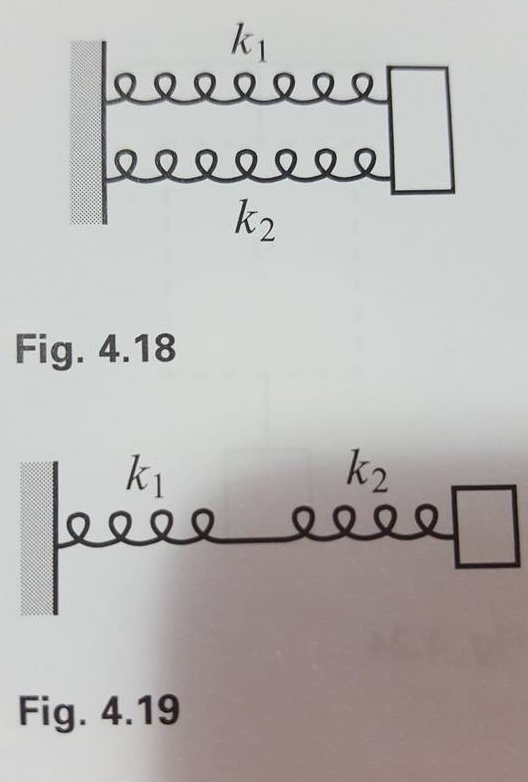# Problem: Two springs with spring constants k1 and k2 are connected in series, as shown in Fig. 4.19. What is the effective spring contant, keff?

###### FREE Expert Solution

Hooke's law:

$\overline{){\mathbf{F}}{\mathbf{=}}{\mathbf{k}}{\mathbf{·}}{\mathbf{∆}}{\mathbf{x}}}$

The springs in series experience the same force. That is:

F = F1 = F2

The displacement of the mass, Δx, is equal to the extension of each sping.

89% (372 ratings)###### Problem Details

Two springs with spring constants kand k2 are connected in series, as shown in Fig. 4.19. What is the effective spring contant, keff?